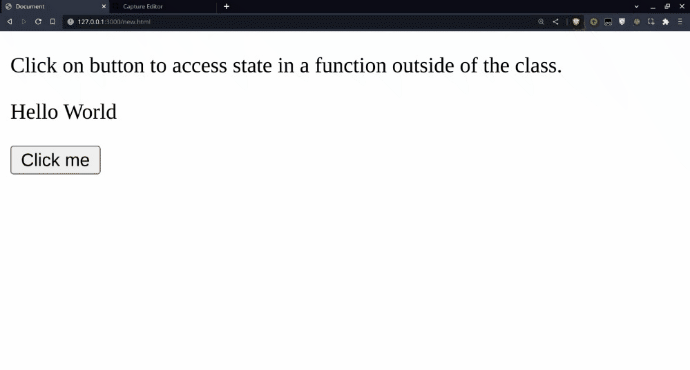Open in App
Not now

# How to access state in a function outside of the class ?

• Last Updated : 02 Feb, 2023

In the context of a function defined outside of a class, state refers to the data associated with an instance of the class being passed as an argument to the function. The function can access and modify this data by referencing the object’s instance variables.

Approach

• Define a class with the desired state as class variables.
• Create an instance of the class.
• Define a function outside of the class.
• Within the function, access the state using the instance name followed by the variable name (i.e. instance_name.variable_name).
• If the function needs to modify the state, pass the instance as an argument and use it to update the variable.
• If the function needs to return the state, return the variable or the instance.
• Use the function and instance to access or update the state as needed.

Example 1: The below example will illustrate the above approach:

## Javascript

 `class MyClass {` `    ``constructor() {` `        ``this``.x = 20;` `    ``}`   `    ``randomMethod() {` `        ``this``.x = 30;` `    ``}` `}`   `function` `randomFunction(obj) {` `    ``console.log(obj.x);` `}`   `const myObj = ``new` `MyClass();` `randomFunction(myObj);` `myObj.randomMethod();` `randomFunction(myObj);`

Output:

```20
30```

The above example shows that randomFunction is defined outside of the MyClass() class. When it is called and passed an instance of MyClass as an argument, it can access the x state of the object (which is initially set to 20). The randomMethod method is then called on the myObj object, which modifies the x state to 30. When randomFunction is called again and passed the modified myObj object as an argument, it outputs the new value of x (30).

Example 2: This the another example showing above approach:

## HTML

 `` `<``html` `lang``=``"en"``>`   `<``head``>` `    ``<``meta` `charset``=``"UTF-8"``>` `    ``<``meta` `http-equiv``=``"X-UA-Compatible"` `          ``content``=``"IE=edge"``>` `    ``<``meta` `name``=``"viewport"` `          ``content``=``"width=device-width, initial-scale=1.0"``>` `    ``<``title``>Document` ``   `<``body``>` `    ``<``p``>Click on button to access state in a ` `      ``function outside of the class.` `    ``<``p` `id``=``"message"``>` `    ``<``button` `id``=``"myBtn"``>Click me` ``   `<``script``>` `    ``let message = "Hello World";` `    ``document.getElementById("message")` `              ``.innerHTML = message;` `    ``const myFunction = (message) => {` `        ``alert(message);` `    ``}`   `    ``document.getElementById("myBtn")` `              ``.addEventListener("click", () => {` `        ``myFunction(message);` `    ``});`   ``   ``

Output:In this example, the myFunction function can access the value of the message variable because it is passed as an argument when the function is called inside the addEventListener function of the button.

This way, you can access the state in a function outside the class.

My Personal Notes arrow_drop_up
Related Articles